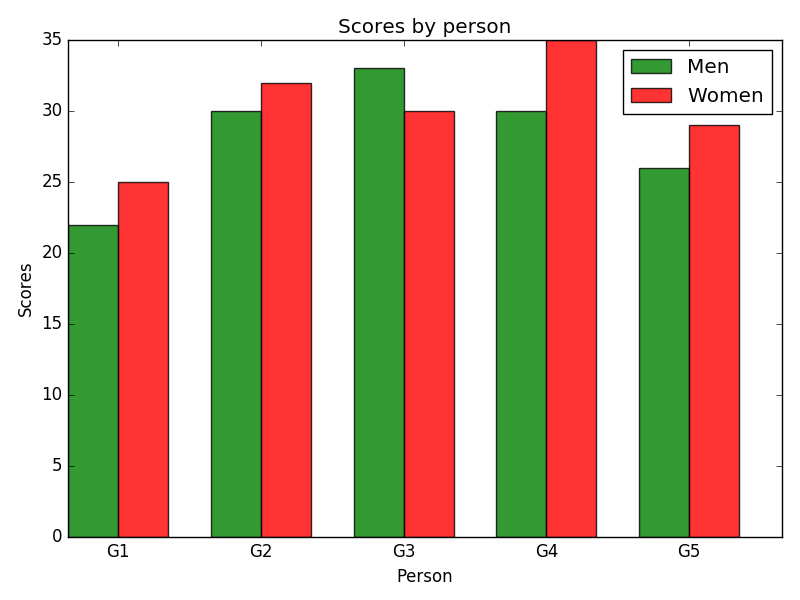﻿ Matplotlib Bar Chart: Create bar plot of scores by group and gender - w3resource# Matplotlib Bar Chart: Create bar plot of scores by group and gender

## Matplotlib Bar Chart: Exercise-10 with Solution

Write a Python program to create bar plot of scores by group and gender. Use multiple X values on the same chart for men and women.

Sample Data:
Means (men) = (22, 30, 35, 35, 26)
Means (women) = (25, 32, 30, 35, 29)

Sample Solution:

Python Code:

``````import numpy as np
import matplotlib.pyplot as plt

# data to plot
n_groups = 5
men_means = (22, 30, 33, 30, 26)
women_means = (25, 32, 30, 35, 29)

# create plot
fig, ax = plt.subplots()
index = np.arange(n_groups)
bar_width = 0.35
opacity = 0.8

rects1 = plt.bar(index, men_means, bar_width,
alpha=opacity,
color='g',
label='Men')

rects2 = plt.bar(index + bar_width, women_means, bar_width,
alpha=opacity,
color='r',
label='Women')

plt.xlabel('Person')
plt.ylabel('Scores')
plt.title('Scores by person')
plt.xticks(index + bar_width, ('G1', 'G2', 'G3', 'G4', 'G5'))
plt.legend()

plt.tight_layout()
plt.show()
```
```

Sample Output:Python Code Editor: